# In a prospective buffer system, as the strength of the weak acid decreases (i.e., as the...

In a prospective buffer system, as the strength of the weak acid decreases (i.e., as the pKa increases), what happens to the strength of the conjugate base?

a. A weak acid has a weak conjugate base.

b. As the strength of the acid decreases, the conjugate base increases in strength.

c. As the strength of the weak acid decreases the strength of the conjugate base decreases as well.

d. It depends on the acid/base system. Some get stronger, some get weaker.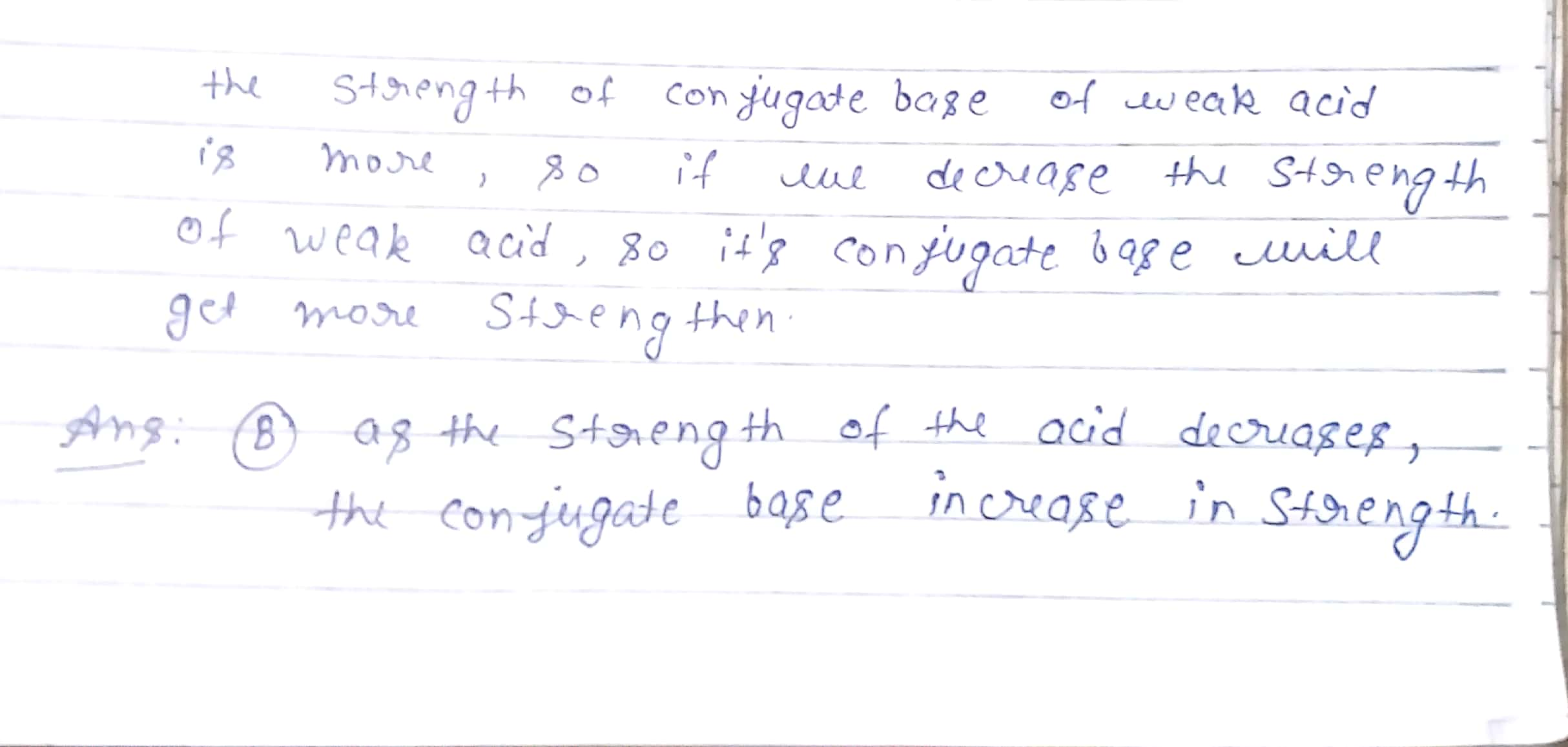#### Earn Coin

Coins can be redeemed for fabulous gifts.

Similar Homework Help Questions
• ### 10. Fill in each blánk with increases or decreases As K, increases, acid strength As Kb...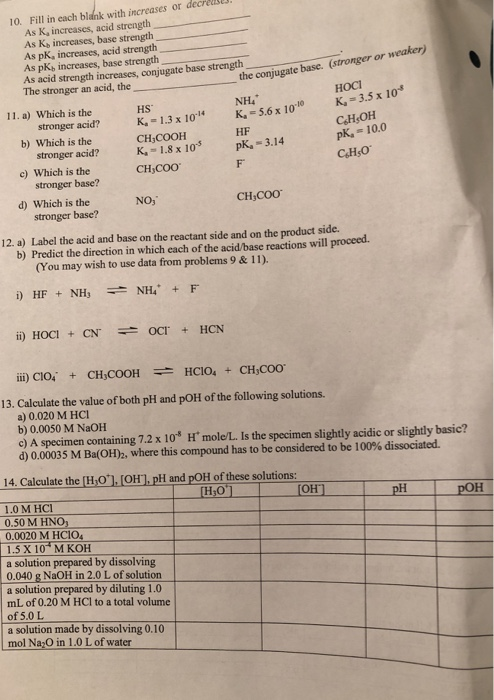10. Fill in each blánk with increases or decreases As K, increases, acid strength As Kb increases, base strength As pK, increases, acid strength As pKb increases, base strength As acid strength increases, conjugate base strength The stronger an acid, the the conjugate base. (stronger or weaker) HoCI NH4 11. a) Which is the HS stronger acid? stronger acid? stronger base? stronger base? HF pk-3.14 CH,OH pk, 10.0 b) Which is the c) Which is the d) Which is the...

• ### A buffer system (pKa = 5) is composed of 0.1 moles of weak acid and 0.05...

A buffer system (pKa = 5) is composed of 0.1 moles of weak acid and 0.05 moles of the conjugate base. What is the pH of the system after the addition of 0.07 moles of a strong base?

• ### Design a buffer that has a pH of 4.77 using one of the weak acid/conjugate base...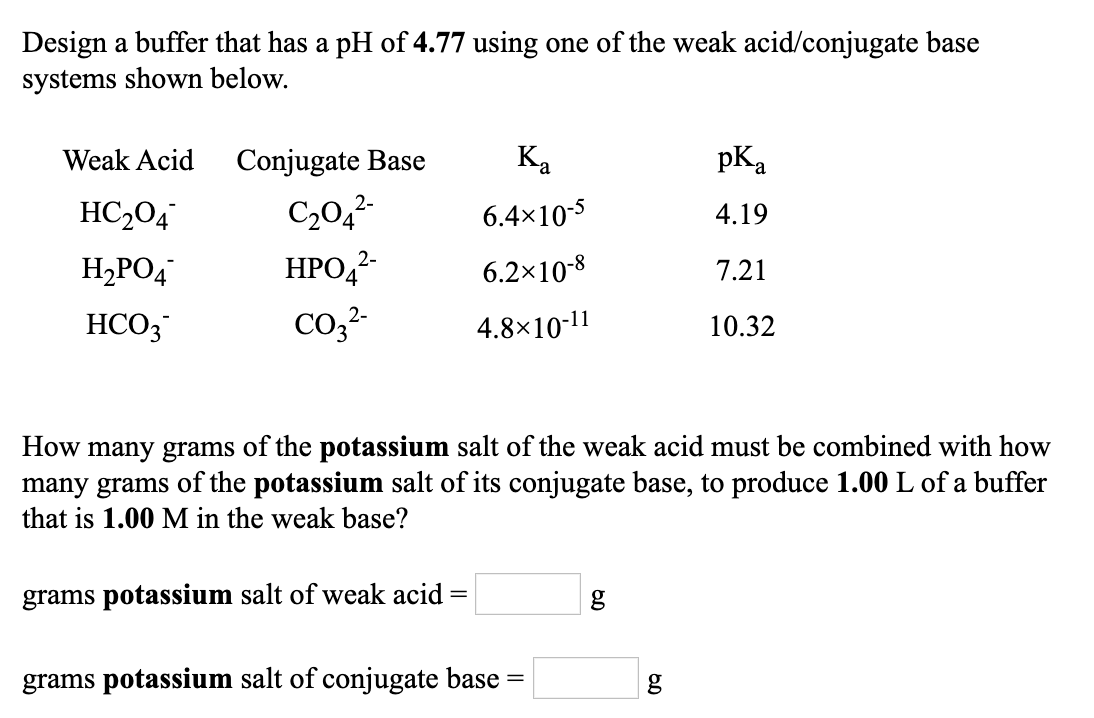Design a buffer that has a pH of 4.77 using one of the weak acid/conjugate base systems shown below. Weak Acid Conjugate Base Ka pKa HC2O4- C2O42- 6.4×10-5 4.19 H2PO4- HPO42- 6.2×10-8 7.21 HCO3- CO32- 4.8×10-11 10.32 How many grams of the potassium salt of the weak acid must be combined with how many grams of the potassium salt of its conjugate base, to produce 1.00 L of a buffer that is 1.00 M in the weak base? grams potassium...

• ### The weak acid of the buffer system is used to prepare the buffer by reacting with...

The weak acid of the buffer system is used to prepare the buffer by reacting with a strong base. The conjugated base is going to be obtained from some of the weak acid in reaction with NaOH 1M. This means you need to calculate the total amount of weak acid needed and the amount of 1.00 M NaOH solution you will have to add to create its conjugate form in solution.   Use 1.0M of each of these acids and basic...

• ### Design a buffer that has a pH of 9.88 using one of the weak base/conjugate acid...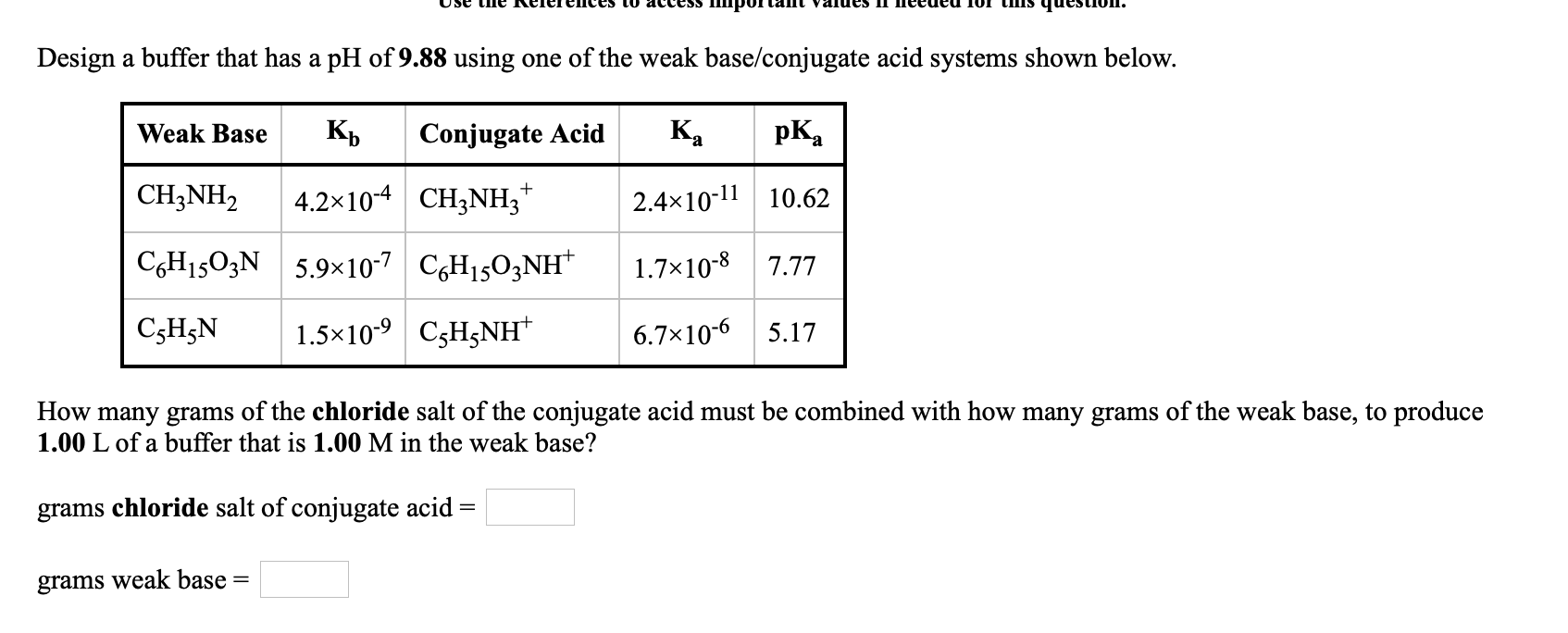Design a buffer that has a pH of 9.88 using one of the weak base/conjugate acid systems shown below. Weak Base Кр Conjugate Acid Ka pKa + CH3NH2 4.2x10-4 CH2NH3 2.4x10-11 10.62 CGH1503N 5.9x10-7 C6H1503NH 1.7x10-8 7.77 C3H5N 1.5x10-9 C3H5NH 6.7x10-6 5.17 How many grams of the chloride salt of the conjugate acid must be combined with how many grams of the weak base, to produce 1.00 L of a buffer that is 1.00 M in the weak base? grams...

• ### a buffer is a solution that has a Weak acid in equlibirum with its conjugate base....

a buffer is a solution that has a Weak acid in equlibirum with its conjugate base. So can a buffer solution has a weak base in equlibirum with its conjugate acid? So basically, to create a buffer, you can mix a weak acid with its conj. base OR a weak acid + strong base OR a weak base with a strong acid? Am i Correct?

• ### A buffer is a solution that is a mixture of either a weak acid and its...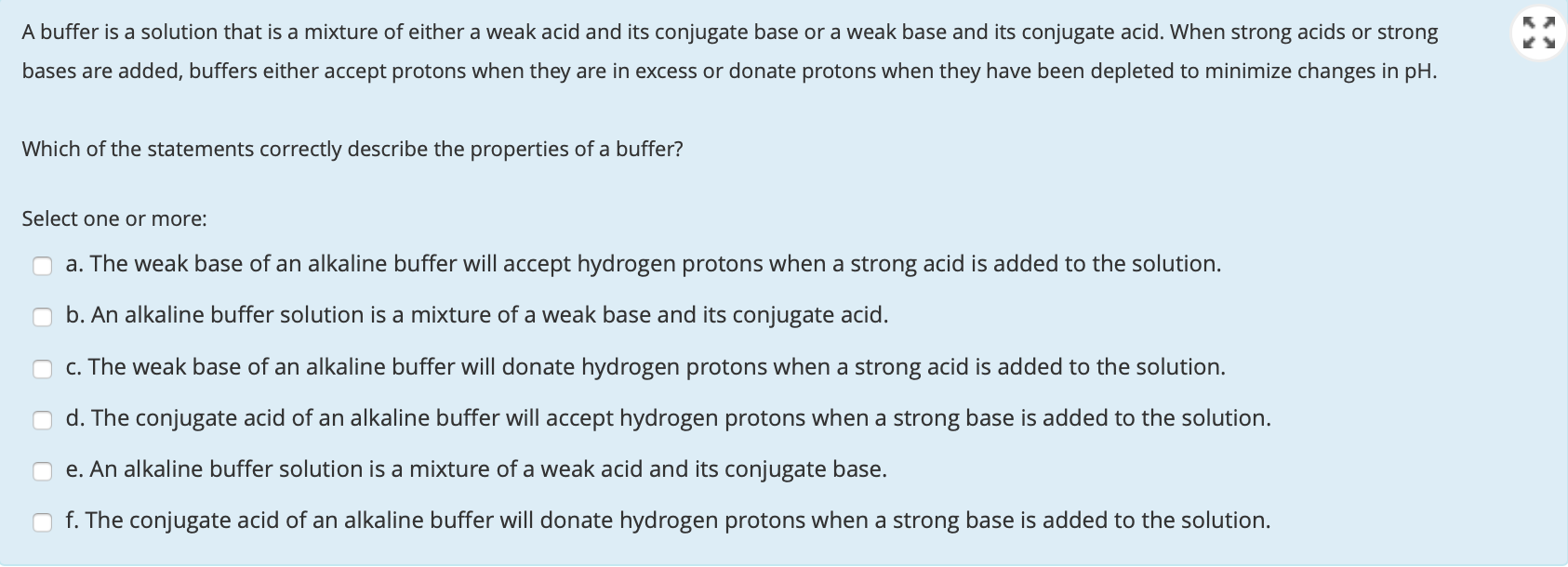A buffer is a solution that is a mixture of either a weak acid and its conjugate base or a weak base and its conjugate acid. When strong acids or strong bases are added, buffers either accept protons when they are in excess or donate protons when they have been depleted to minimize changes in pH. Which of the statements correctly describe the properties of a buffer? Select one or more: a. The weak base of an alkaline buffer will...

• ### Design a buffer that has a pH of 5.85 using one of the weak base/conjugate acid...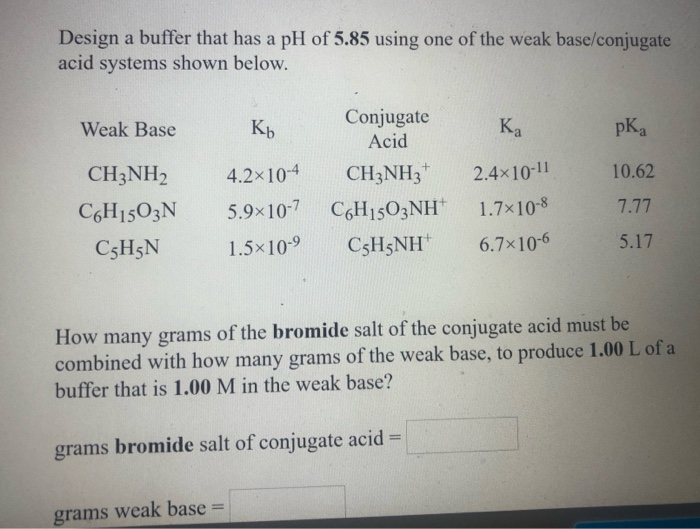Design a buffer that has a pH of 5.85 using one of the weak base/conjugate acid systems shown below. Weak Base Kb pka 10.62 CH3NH2 C6H1503N C3H5N 4.2x10-4 5.9x10-7 1.5*10-9 Conjugate Acid CH3NH2+ CGH1503NH+ CsH5NH ка 2.4x10-11 1.7*10-8 6.7x10-6 7.77 5.17 How many grams of the bromide salt of the conjugate acid must be combined with how many grams of the weak base, to produce 1.00 L of a buffer that is 1.00 M in the weak base? grams bromide...

• ### I DULU LUUAIU 11. 238.011 6. What assumption did you make for the weaker weak acid...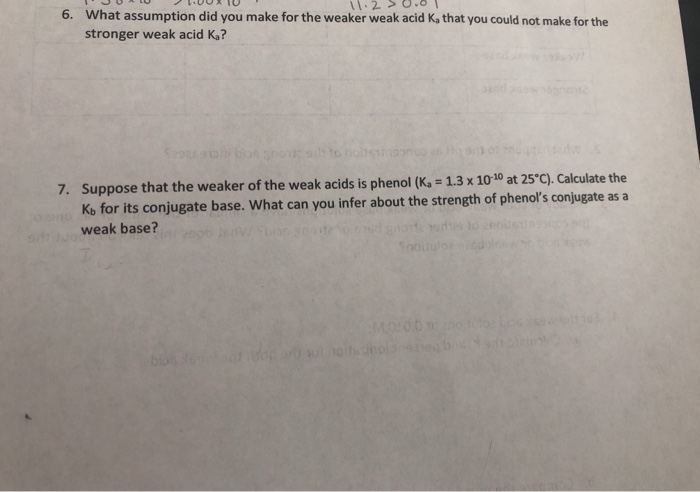I DULU LUUAIU 11. 238.011 6. What assumption did you make for the weaker weak acid Ka that you could not make for the stronger weak acid K? bontott 7. Suppose that the weaker of the weak acids is phenol (K = 1.3 x 10-10 at 25°C). Calculate the Ko for its conjugate base. What can you infer about the strength of phenol's conjugate as a weak base? Shoot

• ### Please answer both 8. If a buffer is prepared with equal volumes of the weak acid...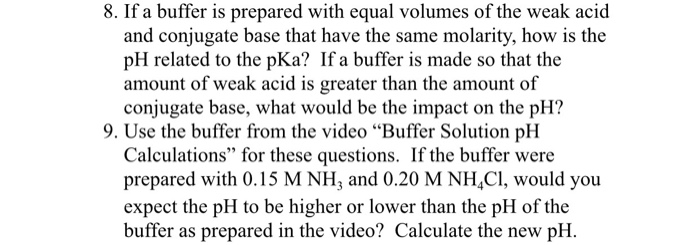Please answer both 8. If a buffer is prepared with equal volumes of the weak acid and conjugate base that have the same molarity, how is the pH related to the pKa? If a buffer is made so that the amount of weak acid is greater than the amount of conjugate base, what would be the impact on the pH? 9. Use the buffer from the video "Buffer Solution pH Calculations'" for these questions. If the buffer were prepared with...

Free Homework App